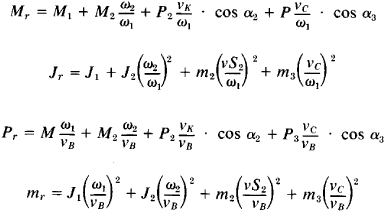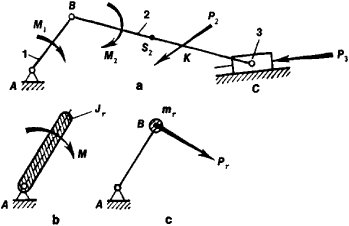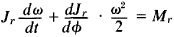# Dynamics of Machines and Mechanisms

The following article is from The Great Soviet Encyclopedia (1979). It might be outdated or ideologically biased.

## Dynamics of Machines and Mechanisms

a branch of the theory of machines and mechanisms that studies the motion of machines and mechanisms, taking into account the forces acting on them. The dynamics of machines and mechanisms deals with the following basic problems: definition of the laws of motion of the components of mechanisms, control of the motion of the components, determination of frictional losses, determination of the reactions in kinematic pairs, and balancing of machines and mechanisms.

The laws of motion of the elements of a mechanism are determined from the given characteristics of external forces using differential equations of motion of a mechanical system or of the machine unit, which usually consists of a motor, a transmission mechanism, the working machine, and in some cases, a control device. The number of equations is equal to the number of degrees of freedom of the mechanical system. For convenience in solving the problem, all forces and masses in plane mechanisms with a single degree of freedom are reduced to a single link or point of the mechanism, which is called the reduction link or reference point. The arbitrary moment applied to the reduction link is called the moment of reduction. The moment of reduction is equal to the aggregate of all moments and forces applied to the links of the mechanism. The arbitrary moment of inertia of the reduction link is called the reduced moment of inertia. The kinetic energy of the reduction link is equal to the sum of the kinetic energies of all links of the mechanism. The reduced force and mass of the mechanism at the point of reduction are determined analogously (Figure 1, a):where Mr is the reduced moment; Jr is the reduced moment of inertia; Pr is the reduced force; mr is the reduced mass; M1, M2, P2, and P3 are the moments and forces applied to theFigure 1. Action of the forces and moments in a crank-and-slide mechanism (a) in the reduction link (b) and at the reference point (c): (1) crank, (2) tie rod, (3) slide, (M) reduced moment Mr, (A) fixed support

links of the mechanism; ω1 and ω2 are the angular velocities of the links; VH and vc are the velocities of points B and C of the mechanism; vS2 is the velocity of the center of gravity of link 2; VK is the velocity of point K of application of the force P2; α2 is the angle between the vectors P2 and VK; and α3 is the angle between the vectors P3 and vc. The equation of motion in this case isthat is, Mr depends in the general case on the time, position, and velocity.

The equations of motion are usually nonlinear. There are no exact methods for the solution of these equations; there-fore, approximate graphic, graphic-analytical, and numerical integration methods are used. The determination of the law of motion of a mechanical system is more difficult if friction and clearances in the kinematic pairs and the variability of the masses of the links are taken into account. In some cases—for example, in the study of transient processes in machines—some of the external forces cannot be considered as fixed, since the motion of the mechanism may have a reverse effect on the characteristics of these forces. For example, under some conditions of high acceleration, it is impossible to take the speed-torque characteristic of an electric motor as consisting of a fixed dependence of the moment on the motor’s shaft on the angular velocity, since electromagnetic processes in the motor may significantly affect the moment. In this case, the differential equation for the electromagnetic processes in the electric motor is added to the differential equations of motion of the mechanical system, and all the equations are solved simultaneously.

Problems of the regulation of the motion of machine assemblies and of their control are treated in control theory. A distinction is made among unsteady, transient, and steady-state conditions of motion. Under steady-state conditions, the velocities of the points of the mechanism are either periodic functions of time or position, or they remain constant. The control of steady-state motion consists in the maintenance of an angular velocity of the reduction link that does not exceed the permissible deviation from its value. A special weight, the flywheel, is designed and installed in the machine for this purpose. The necessity of controlling unsteady motion arises when, in spite of the nonperiodic variation of the external forces or masses, the mean velocity of the reduction link must be maintained at a constant value. Special automatic regulators are installed on the machine for this purpose. In this case, the basic task is the determination of the stability of motion of the machine-regulator system. However, if the velocity or other parameter of any link must be changed in accordance with a fixed law (program), a programming device is installed in the machine. The programmed control of meat-cutting machine tools may serve as an example of this type of control. A concrete problem analyzed by the control theory is the identification of optimum conditions of motion of the machine (optimum control). Examples are the determination of the motion involving the fastest transient regime at a limited acceleration (the optimum motion with respect to the speed of operation) or of the motion involving a minimum of energy expenditure in the transient regime (the optimum motion with respect to losses).

The determination of losses other than working losses in machines reduces to the determination of frictional losses, which are basic in nature and affect the efficiency of machines and mechanisms. The degree of utilization of energy in a machine is estimated in terms of the mechanical efficiency.

The kinetostatic calculations involving mechanisms, which are performed for known conditions of motion of the mechanism, are performed by determining the reactions in kinematic pairs to all the given external forces, as well as to the inertial forces of the links and the frictional forces in the kinematic pairs. The values of these reactions are part of the calculation of the strength of the links and are required for the selection of bearings and for the calculation of their lubrication.

Machines and mechanisms are balanced by a rational selection and placement of counterweights, which reduce the dynamic pressures in the kinematic pairs of mechanisms. In practice, machines are stabilized by mounting on a foundation (prevention of vibrations) or by balancing the rotating masses. The inertial forces in modern high-speed machines attain high values. The forces of inertia, which vary in magnitude and direction, disrupt the normal operations of the elements of the machine and are sources of vibrations and noise, which adversely affect the operating personnel and prevent the normal functioning of other mechanisms and devices. In vibration machines, the conditions for the generation of intense vibrations in their working elements are taken into account. Dynamic studies in machines are directly related to the calculation of the strength and stiffness of machine elements, which are performed in connection with the selection of the dimensions and the design of parts. Methods for such calculations are usually described in training disciplines, such as strength of materials, the dynamics of structures, and machine parts.

Dynamic studies are also performed for three-dimensional mechanisms with many degrees of freedom. The operations performed by systems of this type have a high degree of universality.

### REFERENCES

Kožešnik, J. Dinamika mashin. Moscow, 1961. (Translated from Czech.)
Zinov’eva, V. A., and A. P. Bessonov. Osnovy dinamiki mashinnykh agregatov. Moscow, 1964.
Artobolevskii, I. I. Teoriia mekhanizmov, 2nd ed. Moscow, 1967.
Kozhevnikov, S. N. Teoriia mekhanizmov i mashin, 3rd ed. Moscow, 1969.

I. I. ARTOBOLEVSKII and A. P. BESSONOV

The Great Soviet Encyclopedia, 3rd Edition (1970-1979). © 2010 The Gale Group, Inc. All rights reserved.
Site: Follow: Share:
Open / Close